# Scales

“Scales are functions that map from an input domain to an output range.”

The values in any data set are unlikely to correspond exactly to pixel measurements for use in your visualization. Scales provide a convenient way to map those data values to new values useful for visualization purposes.

D3 scales are functions whose parameters you define. Once they are created, you call the scale function, pass it a data value, and it nicely returns a scaled output value. You can define and use as many scales as you like.

It may be tempting to think of a scale as something that appears visually in the final image — like a set of tick marks, indicating a progression of values. Do not be fooled! Those tick marks are part of an axis, which is essentially a visual representation of a scale. A scale is a mathematical relationship, with no direct visual output. I encourage you to think of scales and axes as two different, yet related, elements.

This topic addresses only linear scales, since they are most common and understandable. Once you understand linear scales, the others will be a piece of cake.

## Apples and Pixels

Imagine that the following data set represents the number of apples sold at a roadside fruit stand each month:

```var dataset = [ 100, 200, 300, 400, 500 ];

```

First of all, this is great news, as the stand is selling 100 additional apples each month! Business is booming. To showcase this success, you want to make a bar chart illustrating the steep upward climb of apple sales, with each data value corresponding to the height of one bar.

Until now, we’ve used data values directly as display values, ignoring unit differences. So if 500 apples were sold, the corresponding bar would be 500 pixels tall.

That could work, but what about next month, when 600 apples are sold? And a year later, when 1,800 apples are sold? Your audience would have to purchase ever-larger displays, just to be able to see the full height of those very tall apple-bars! (Mmm, apple bars!)

This is where scales come in. Because apples are not pixels (which are also not oranges), we need scales to translate between them.

## Domains and Ranges

A scale’s input domain is the range of possible input data values. Given the apples data above, appropriate input domains would be either 100 and 500 (the minimum and maximum values of the data set) or zero and 500.

A scale’s output range is the range of possible output values, commonly used as display values in pixel units. The output range is completely up to you, as the information designer. If you decide the shortest apple-bar will be 10 pixels tall, and the tallest will be 350 pixels tall, then you could set an output range of 10 and 350.

For example, create a scale with an input domain of `100,500` and an output range of `10,350`. If you gave that scale the value `100`, it would return `10`. If you gave it `500`, it would spit back `350`. If you gave it `300`, it would hand `180` back to you on a silver platter. (`300` is in the center of the domain, and `180` is in the center of the range.)

We could visualize the domain and range as corresponding axes, side-by-side:

One more thing: Given that it is very easy to mix up the input domain and output range terminology, I’d like to propose a little exercise. When I say “input,” you say “domain.” Then I say “output,” and you say “range.” Ready? Okay:

• Input! Domain!
• Output! Range!
• Input! Domain!
• Output! Range!

Got it? Great.

## Normalization

If you’re familiar with the concept of normalization, it may be helpful to know that, with a linear scale, that’s all that is really going on here.

Normalization is the process of mapping a numeric value to a new value between 0 and 1, based on the possible minimum and maximum values. For example, with 365 days in the year, day number 310 maps to about 0.85, or 85% of the way through the year.

With linear scales, we are just letting D3 handle the math of the normalization process. The input value is normalized according to the domain, and then the normalized value is scaled to the output range.

## Creating a Scale

D3’s scale generators are accessed with `d3.scale` followed by the type of scale you want.

```

var scale = d3.scaleLinear();

```

Congratulations! Now `scale` is a function to which you can pass input values. (Don’t be misled by the `var` above; remember that in JavaScript, variables can store functions.)

```

scale(2.5);  //Returns 2.5

```

Since we haven't set a domain and a range yet, this function is mapping input to output on a 1:1 scale. That is, whatever we input will be returned unchanged.

We can set the scale’s input domain to `100,500` by passing those values to the `domain()` method as an array:

```

scale.domain([100, 500]);

```

Set the output range in similar fashion, with `range()`:

```

scale.range([10, 350]);

```

These steps can be done separately, as above, or chained together into one line of code:

```

var scale = d3.scaleLinear()

.domain([100, 500])
.range([10, 350]);

```

Either way, our scale is ready to use!

```

scale(100);  //Returns 10

scale(300);  //Returns 180
scale(500);  //Returns 350

```

Typically, you will call scale functions from within an `attr()` method or similar, not on their own. Let’s modify our scatterplot visualization to use dynamic scales.

## Scaling the Scatterplot

```

var dataset = [

[5, 20], [480, 90], [250, 50], [100, 33], [330, 95],
[410, 12], [475, 44], [25, 67], [85, 21], [220, 88]
];

```

You’ll recall that `dataset` is an array of arrays. We mapped the first value in each array onto the x axis, and the second value onto the y axis. Let’s start with the x axis.

Just by eyeballing the x values, it looks like they range from 5 to 480, so a reasonable input domain to specify might be `0,500`, right?

Why are you giving me that look? Oh, because you want to keep your code flexible and scalable, so it will continue to work even if the data change in the future. Very smart!

Instead of specifying fixed values for the domain, we can use convenient array functions like `min()` and `max()` to analyze our data set on the fly. For example, this loops through each of the x values in our arrays and returns the value of the greatest one:

```

d3.max(dataset, function(d) {    //Returns 480

return d;  //References first value in each sub-array
});

```

Putting it all together, let’s create the scale function for our x axis:

```

var xScale = d3.scaleLinear()

.domain([0, d3.max(dataset, function(d) { return d; })])
.range([0, w]);

```

First, notice I named it `xScale`. Of course, you can name your scales whatever you want, but a name like `xScale` helps me remember what this function does.

Second, notice I set the low end of the input domain to zero. (Alternatively, you could use `min()` to calculate a dynamic value.) The upper end of the domain is set to the maximum value in `dataset` (which is 480).

Finally, observe that the output range is set to `0` and `w`, the SVG’s width.

We’ll use very similar code to create the scale function for the y axis:

```

var yScale = d3.scaleLinear()

.domain([0, d3.max(dataset, function(d) { return d; })])
.range([0, h]);

```

Note that the `max()` function references `d`, the y value of each sub-array. Also, the upper end of `range()` is set to `h` instead of `w`.

The scale functions are in place! Now all we need to do is use them. Simply modify the code where we create a `circle` for each data value

```

.attr("cx", function(d) {

return d;
})

```

to return a scaled value (instead of the original value):

```

.attr("cx", function(d) {

return xScale(d);
})

```

Likewise, for the y axis, this

```

.attr("cy", function(d) {

return d;
})

```

is modified as:

```

.attr("cy", function(d) {

return yScale(d);
})

```

For good measure, let’s make the same change where we set the coordinates for the text labels, so these lines

```

.attr("x", function(d) {

return d;
})
.attr("y", function(d) {
return d;
})

```

become this:

```

.attr("x", function(d) {

return xScale(d);
})
.attr("y", function(d) {
return yScale(d);
})

```

And there we are!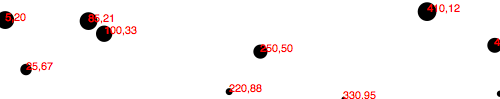Here’s the working code. Visually, it is disappointingly similar to our original scatterplot! Yet we are making more progress than may be apparent.

## Refining the Plot

You may have noticed that smaller y values are at the top of the plot, and the larger y values are toward the bottom. Now that we’re using scales, it’s super easy to reverse that, so greater values are higher up, as you would expect. It’s just a matter of changing the output range of `yScale` from

```

.range([0, h]);

```

to

```

.range([h, 0]);

```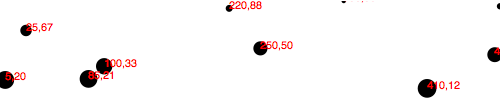Here’s that code. Yes, now a smaller input to `yScale` will produce a larger output value, thereby pushing those `circle`s and `text` elements down, closer to the base of the image. I know, it’s almost too easy!

Yet some elements are getting cut off. Let’s introduce a `padding` variable:

```

```

Then we’ll incorporate the `padding` amount when setting the range of both scales. The range for `xScale` was `range([0, w])`, but now it’s

```

```

The range for `yScale` was `range([h, 0])`, but now it’s

```

```

This should provide us with 20 pixels of extra room on the left, right, top, and bottom edges of the SVG. And it does!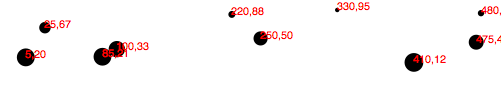But the text labels on the far right are still getting cut off, so I’ll double the amount of `xScale`’s padding on the right side by multiplying by two:

```

```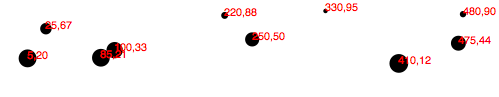Better! Here’s the code so far. But there’s one more change I’d like to make. Instead of setting the radius of each `circle` as the square root of its y value (which was a bit of a hack, and not useful in any case), why not create another custom scale?

```

var rScale = d3.scaleLinear()

.domain([0, d3.max(dataset, function(d) { return d; })])
.range([2, 5]);

```

Then, setting the radius looks like this:

```

.attr("r", function(d) {

return rScale(d);
});

```

This is exciting, because we are guaranteeing that our radius values will always fall within the range of `2,5`. (Or almost always: See reference to `clamp()` below.) So data values of `0` (the minimum input) will get circles of radius `2` (or a diameter of 4 pixels). The very largest data value will get a circle of radius `5` (diameter of 10 pixels).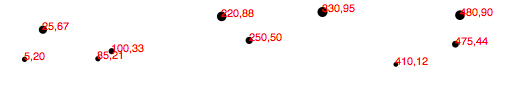Voila: Our first scale used for a visual property other than an axis value.

Finally, just in case the power of scales hasn’t yet blown your mind, I’d like to add one more array to the data set: `[600, 150]`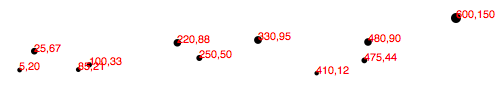Boom! Here’s the code. Notice how all the old points maintained their relative positions, but have migrated closer together, down and to the left, to accommodate the newcomer.

And now, one final revelation: We can now very easily change the size of our SVG, and everything scales accordingly. Here, I’ve increased the value of `h` from `100` to `300` and made no other changes: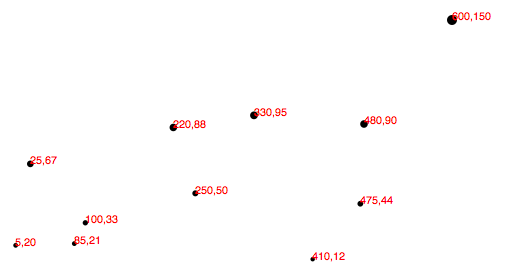Boom, again! Here’s the updated code. Hopefully, you are seeing this and realizing: No more late nights tweaking your code because the client decided the graphic should be 800 pixels wide instead of 600. Yes, you will get more sleep because of me (and D3’s brilliant built-in methods). Being well-rested is a competitive advantage. You can thank me later.

## Other Methods

`d3.scaleLinear()` has several other handy methods that deserve a brief mention here:

• `nice()` — This tells the scale to take whatever input domain that you gave to `range()` and expand both ends to the nearest round value. From the D3 documentation: “For example, for a domain of [0.20147987687960267, 0.996679553296417], the nice domain is [0.2, 1].” This is useful for normal people, who find it hard to read numbers like 0.20147987687960267.
• `rangeRound()` — Use `rangeRound()` in place of `range()` and all values output by the scale will be rounded to the nearest whole number. This is useful if you want shapes to have exact pixel values, to avoid the fuzzy edges that may arise with antialiasing.
• `clamp()` — By default, a linear scale can return values outside of the specified range. For example, if given a value outside of its expected input domain, a scale will return a number also outside of the output range. Calling `.clamp(true)` on a scale, however, forces all output values to be within the specified range. Meaning, excessive values will be rounded to the range’s low or high value (whichever is nearest).

## Other Scales

In addition to `linear` scales (discussed above), D3 has several other scale methods built-in:

Next up: Axes